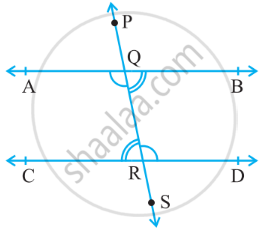# If a Transversal Intersects Two Parallel Lines, Then Each Pair of Alternate Interior Angles Are Equal.Given: Two parallel lines AB and CD.

Let PS be the transversal intersecting AB at Q and CD at R.

To Prove: Each pair of alternate interior angles are equal.

i.e., ∠BQR = ∠ CRQ

and ∠ AQR = ∠ QRD.

Proof:

First, we will prove ∠ BQR = ∠ CRQ.

For lines AB & CD, with transversal PS.

∠ AQP = ∠ CRQ    .....(Corresponding angles)(1)

For lines AB & PS,

∠ AQP = ∠ BQR    ......(Vertically opposite angles)(2)

From (1) and (2),

∠ BQR = ∠ CRQ

Similarly, we can prove

∠ AQR = ∠ QRD

Hence, Pair of alternate interior angles are equal.

Hence proved.

If you would like to contribute notes or other learning material, please submit them using the button below.

### Shaalaa.com

Axiom: If a Transversal Intersects Two Parallel Lines, Then Each Pair of Alternate Interior Angles Are Equal. [00:05:30]
S
0%